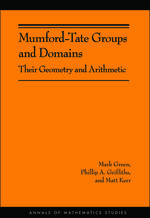# Mumford-Tate Groups and Domains: Their Geometry and Arithmetic (AM-183)

Mark Green
Phillip Griffiths
Matt Kerr
Pages: 256
https://www.jstor.org/stable/j.ctt7s3h1

1. Front Matter
(pp. i-iv)
(pp. v-viii)
3. Introduction
(pp. 1-27)

Mumford-Tate groups are the fundamental symmetry groups in Hodge theory. They were introduced in the papers [M1] and [M2] by Mumford. As stated there the purpose was to interpret and extend results of Shimura and Kuga ([Sh1], [Sh2], and [Ku]). Since then they have played an important role in Hodge theory, both in the formal development of the subject and in the use of Hodge theory to address algebro-geometric questions, especially those that are arithmetically motivated. The informative sets of notes by Moonen [Mo1] and [Mo2] and the recent treatment in [PS] are two general accounts of the subject.

We...

4. Chapter I Mumford-Tate Groups
(pp. 28-44)

LetVbe a$\mathbb{Q}$-vector space. For$k = \mathbb{R}$or$\mathbb{C}$we set$V_k = V \otimes k$and GL(V)k= GL(Vk). There are three definitions of aHodge structure of weight n, here given in historical order. In the first two definitions, we assume thatnis positive and thep, q’s in the definitions are non-negative. In the third definition, which will be the one used primarily in this monograph,nandp, qare arbitrary integers.

Definition (i): AHodge structure of weight nis given by aHodge decomposition

$\left\{ {\begin{array}{*{20}c} {V_\mathbb{C} = \mathop \otimes \limits_{p + q = n} V^{p,q} } \\ {V^{q,p} = \bar V^{p,q} .} \\ \end{array} } \right.$

Definition (ii): AHodge structure of weight nis given...

5. Chapter II Period Domains and Mumford-Tate Domains
(pp. 45-66)

The principal general reference is [C-MS-P]. We refer also to [CGG] for a survey and some more recent developments. We assume given (V,Q) as in Section I.A, together with a set ofHodge numbers hp,n—p=hn—p,pwith$\Sigma _{p = 0}^n h^{p,n - p}$= dimV. We also set$f^p = \Sigma _{p'} _{ \geqq p} h^{p',n - p'}$.

Definition: Theperiod domain Dassociated to the above data is the set of polarized Hodge structures (V, Q,φ) with dimVp,q=hp,q.

The natural symmetry group acting onDis the groupG($\mathbb{R}$) of real points of the$\mathbb{Q}$-algebraic groupG= Aut(V,Q). Elementary linear algebra...

6. Chapter III The Mumford-Tate Group of a Variation of Hodge Structure
(pp. 67-84)

Let$\Gamma \subset G$be a discrete subgroup of$G(\mathbb{R})$. It acts properly discontinuously onDand the quotient

$\cal{M}_\Gamma = \Gamma \backslash D$

is a complex analytic variety. It is smooth outside of the images of the fixed points of Γ; locally these are quotients of a polycylinder in$\mathbb{C}^N$by a finite group—i.e., they arequotient singularities. We shall refer to$\cal{M}_\Gamma$as themoduli space ofΓ-equivalence classes of polarized Hodge structures.

Definition: Avariation of Hodge structure(VHS) is given by

(III.1)

$\Phi :S \to \cal{M}_\Gamma$

whereSis a connected complex manifold and$\Phi$is a locally liftable, holomorphic mapping that is...

7. Chapter IV Hodge Representations and Hodge Domains
(pp. 85-186)

Blanket assumption for this chapter: M will be asemi-simple$\mathbb{Q}$-algebraic group. In this case, the Lie algebra m is a direct sum${\text{m}} = \oplus {\text{m}}_i$where the miare simple Lie algebras over$\mathbb{Q}$. The groupMis then an almost direct product of the$\mathbb{Q}$-simple algebraic groupsMiwhose Lie algebra is mi[Bor].¹

The basic questions underlying this section are

Which M can be Mumford-Tate groups of polarized Hodge structures?and, more fundamentally,

What can one say about the different realizations of M as a Mumford-Tate group?

What can one say about the corresponding Mumford-Tate domains?...

8. Chapter V Hodge Structures with Complex Multiplication
(pp. 187-212)

This largely expository chapter deals with Hodge structures having “lots” of endomorphisms — so many Hodge 2-tensors that the Mumford-Tate group is forced inside the isotropy group, or equivalently:

(V.1) A Hodge structure (V,φ) hascomplex multiplication, oris a CM-Hodge structure, if and only ifMφis a torus.

CM-Hodge structures will play a pivotal role in Chapters VI–VII as convenient “base points” in period domains, or as one-point period domains, and in Chapter VIII as a focal point for conjectures on the arithmetic properties of variation of Hodge structure. They generalize isogeny classes of CM abelian...

9. Chapter VI Arithmetic Aspects of Mumford-Tate Domains
(pp. 213-239)

We start by remarking on a few points concerning the structure and construction of Mumford-Tate domains. Let φ be aQ-polarized Hodge structure onVwith Mumford-Tate group

$M = M_\varphi \subseteq G: = Aut(V,Q)^0 ,$

andHφ(resp.Pφ) the stabilizer of φ (resp.$F_\phi ^ \bullet$) inG. The algebraic groupsMφ,Hφ, andPφare connected,¹ closed, and reductive.

(VI.1) Lemma:MφHφandMφPφare connected (as algebraic groups).

Proof. We doHφ; proof forPφis nearly identical. Suppose otherwise, and write$M_\varphi \cap H_\varphi = Z_0 \coprod {\coprod\limits_{i = 1}^m {Z_i } }$, with$Z_1^k \subset Z_0$, and whereZ0, and whereZ0denotes the identity component. Any element$\alpha \in Z_1 (\mathbb{C})$is...

10. Chapter VII Classification of Mumford-Tate Subdomains
(pp. 240-268)

In this chapter we will produce an algorithm for deducing all Mumford-Tate subdomains of a given period domain—or equivalently, all possible Mumford-Tate groups of polarized Hodge structure with given Hodge numbers. We will then apply it in the case of rank 4 Hodge structures of weight 1 (Hodge numbers (2, 2)) and weight 3 (Hodge numbers (1, 1, 1, 1)), working out as much data as possible via the techniques of Chapter VI — Hodge tensors, endomorphism algebra, number of components in the Mumford-Tate Noether-Lefschetz loci (inDresp.Ď), and so forth.

The key motivating idea for our...

11. Chapter VIII Arithmetic of Period Maps of Geometric Origin
(pp. 269-276)

Recall that a period domainDhas a differential ideal$\cal{J} \subset \Omega ^ \bullet (D)$that must pull back to zero under the local lifting of any VHS. We refer to$\cal{J}$as the infinitesimal period relation (IPR). A period domain on which$\cal{J}$is zero, is calledunconstrained. More generally, a Mumford-Tate domain on which the pullback of$\cal{J}$is zero is also calledunconstrained. A key point is that the quotients, by arithmetic groups Γ, of unconstrained period and Mumford-Tate domains, including unconstrained Mumford-Tate subdomains of constrained period domains, will yield quasi-projective (Shimura¹) varieties, with the projective embedding given by automorphic functions.²...

12. Bibliography
(pp. 277-286)
13. Index
(pp. 287-289)